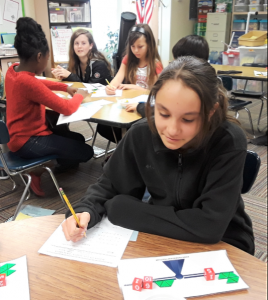# The Hands-On Equations Fractions Learning System

Leave a Review

\$29.50

This is the complete Hands-On Equations Fractions program for use with one student. Includes manuals for Levels I and II, worksheets, answer key, and one student kit of game pieces consisting of a flat laminated balance, a set of eight number cubes, and a set of 33 fraction blocks.

SKU: HF-1000-P

## Description

### Methodology

This Hands-On Equations Fractions program consists of two levels encompassing a total of 12 lessons. As with the original Hands-On Equations program, students represent the fractional equation on a flat laminated scale using their game pieces, and then use “legal moves” to solve the equations. Each lesson comes with a worksheet containing 8 problems.

Unique to the Hands-On Equations Fractions approach, students solve first for the unit fraction of the unknown. Hence, for example, if the blue block representing a third of the unknown y is found to have a value of 4, they can figure out that the yellow block, representing the unknown y, has a value of 12.  This methodology provides students with a sense-making approach to fractional linear equations.

Each level ends with an abstract solution approach which may be presented to younger students as an enrichment lesson. For middle or high school students, it is important that the teacher lead them to solutions using only paper and pencil.There are no reviews yet.

Only logged in customers who have purchased this product may leave a review.